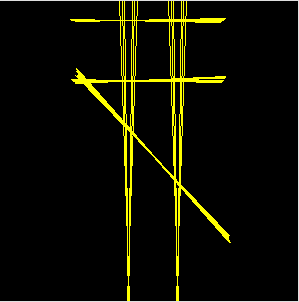## Calculating Euclidean Rhythmns using the Bresenham Algorithm

Recently I started playing with euclidean rhythms and implemented the bjorklund algorithm shown in the paper by Godfired Toussaint in ruby. The implementation isn't really rocket science (actually it's ring accelerator science :-)) but it uses recursive function calls, two arrays, multiple stages, ... and I had the feeling that this is far more complicated than it needs to be. After reading several posts, blogs and papers about the implementations of the algorithm I read somewhere that the algorithm produces the same result as the bresenham algorithm - at first I ignored the sentence and unfortunately I have no idea anymore where I read it but today I implemented a simple version of it an the results I get are the same as my recursive version - or rotations, which is fine because you usually loop over the sequences and are free to choose a starting point - so in case anyone is interested here is my bresenhamish method for calculating euclidean rhythms in ruby - shouldn't be too complicated to port it to different languages

``````def eucledean(k,n)
f = 1.0*n/k
res = Array.new(n,0);
a = 0
k.times do |i|
res[a.to_i] = 1
a += f
end
return res
end

(1..8).each do |i|
print eucledian(i,8)
print "\n"
end
``````

and this are the results I get for the 8 possible rhythms with 8 steps

``````[1, 0, 0, 0, 0, 0, 0, 0]
[1, 0, 0, 0, 1, 0, 0, 0]
[1, 0, 1, 0, 0, 1, 0, 0]
[1, 0, 1, 0, 1, 0, 1, 0]
[1, 1, 0, 1, 1, 0, 1, 0]
[1, 1, 1, 0, 1, 1, 1, 0]
[1, 1, 1, 1, 1, 1, 1, 0]
[1, 1, 1, 1, 1, 1, 1, 1]
``````

## hexagonal labyrinths in processing

I took my labyrinth generator and reworked the algorithm a bit to generate hexagonal labyrinths.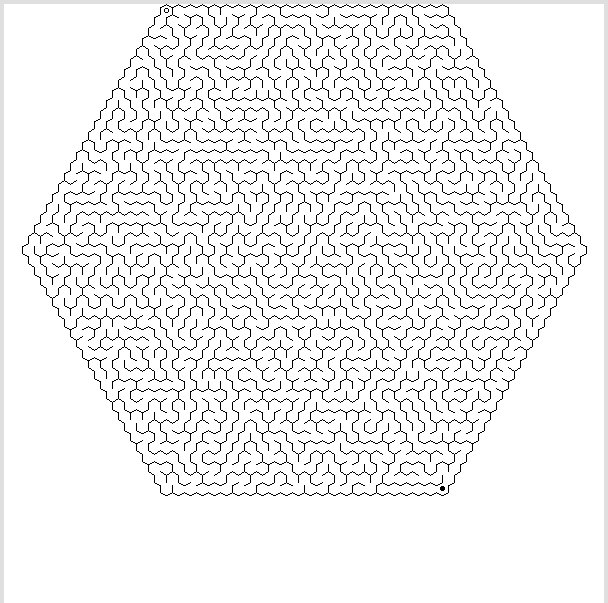## Generating Labyrinths in Processing

I wrote a processing-sketch that generates labyrinths. It uses a simple recursive algorithm described here to generate the maze.

The + and - keys can be used to change the maze-size, any other key generates a new maze.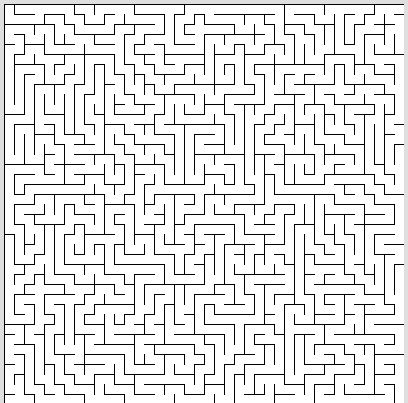## line detection with hough-transform

I wrote a small processing sketch to work out how linedetection using hough-transform works.

click here to see it in action and get the sourcecode. the code is not optimised for speed but for my understanding :-) so don't complain if it doesn't work on live-video.

the algorithm takes a image that has run through an edge detection algorithm and turned into a black/white image and find where things like edges or circles are by converting the pixels from imagespace to a parameterspace where such detection is much easyer.

for each pixel that is set in the source image a set of parameters that satisfy the formular r = x * cos(roh) + y * sin(roh) is calculated and r and roh are plotted as the parameterspace image

every line in the input image results in a bunch of lines in the parameterspace that have a common intersection point.

in my example i use a input image like this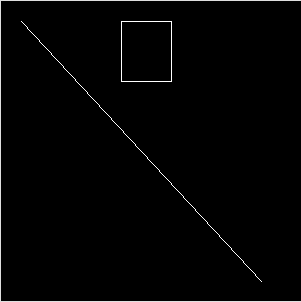calculate how it looks in parameter space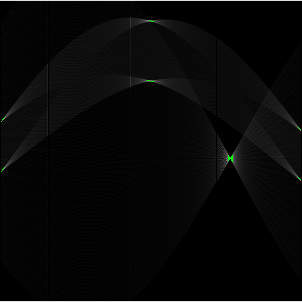and then reconstruct where the lines are